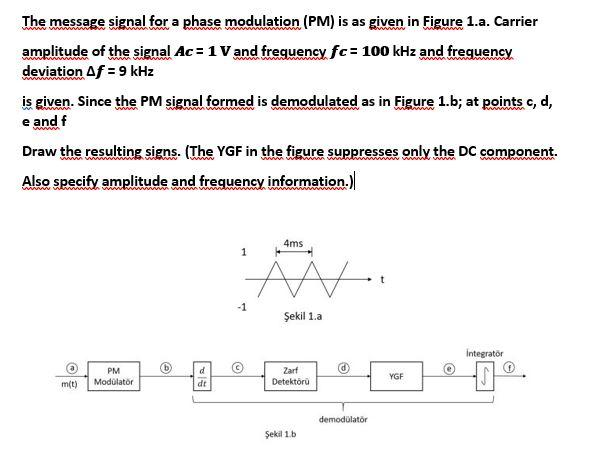# Question Solved1 AnswerThe message signal for a phase modulation (PM) is as given in Figure 1.a. Carrier amplitude of the signal Ac = 1 V and frequency fc= 100 kHz and frequency wwwwww deviation Af = 9 kHz is given. Since the PM signal formed is demodulated as in Figure 1.b; at points c, d, e and f Draw the resulting signs. (The YGF in the figure suppresses only the DC component. Also specify amplitude and frequency information.) 4ms 1 M V Şekil 1.a Integrator © e 12 PM Modolator Zarf Detektoru YGF m(t) dt demodulator Şekil 1bRMUZXO The Asker · Electrical EngineeringTranscribed Image Text: The message signal for a phase modulation (PM) is as given in Figure 1.a. Carrier amplitude of the signal Ac = 1 V and frequency fc= 100 kHz and frequency wwwwww deviation Af = 9 kHz is given. Since the PM signal formed is demodulated as in Figure 1.b; at points c, d, e and f Draw the resulting signs. (The YGF in the figure suppresses only the DC component. Also specify amplitude and frequency information.) 4ms 1 M V Şekil 1.a Integrator © e 12 PM Modolator Zarf Detektoru YGF m(t) dt demodulator Şekil 1b
More
Transcribed Image Text: The message signal for a phase modulation (PM) is as given in Figure 1.a. Carrier amplitude of the signal Ac = 1 V and frequency fc= 100 kHz and frequency wwwwww deviation Af = 9 kHz is given. Since the PM signal formed is demodulated as in Figure 1.b; at points c, d, e and f Draw the resulting signs. (The YGF in the figure suppresses only the DC component. Also specify amplitude and frequency information.) 4ms 1 M V Şekil 1.a Integrator © e 12 PM Modolator Zarf Detektoru YGF m(t) dt demodulator Şekil 1b